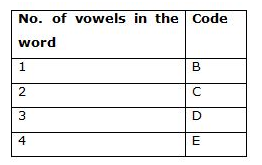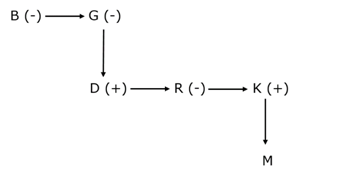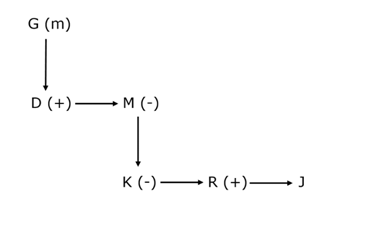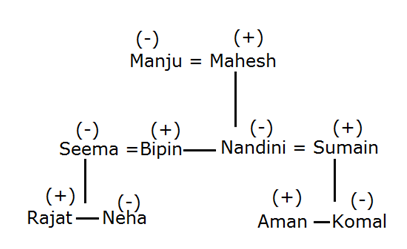# SBI Clerk Mains 2021 Reasoning Ability (Day-04)

Dear Aspirants, Our IBPS Guide team is providing new series of Reasoning Questions for SBI Clerk Mains 2021 so the aspirants can practice it on a daily basis. These questions are framed by our skilled experts after understanding your needs thoroughly. Aspirants can practice these new series questions daily to familiarize with the exact exam pattern and make your preparation effective.

Start Quiz

Coding decoding

Direction (1-3): Study the following information carefully and answers the questions given below:

In a certain code language,

“Lynch Called Songs” is coded as “S25Z      B19O    C12M”.

“Rhythm Twenty Identical” is coded as “N25I   E20F   B25F”

“System Together Celebrate” is coded as “D20H    B25T      E20M”.

1) What is the code for ‘Simplify’?

A.D21L

B.C25L

C.C25N

D.B22L

E.None of these

2) What is the code for ‘Hymn’?

A.N25Z

B.Z25M

C.X23H

D.M25Z

E.M23Z

3) What is the code for ‘Announced’?

A.E21O

B.E21M

C.D21M

D.B20M

E.None of these

Coded blood relation

Directions (4-5): Study the following information carefully and answer the questions given below it.

‘A @ B’ means ‘A is the father of B’.

‘A % B’ means ‘A is the brother of B’.

‘A # B’ means ‘A is the mother of B’.

‘A & B’ means ‘A is the sister of B’.

4) In the given statement “B&G#D%R&[email protected]”, how G is related to M?

A.Aunty

B.Maternal Grandfather

C.Father-in-law

D.Grandmother

E.Maternal Aunty

5) Which of the following option correctly fill in the blanks in thegiven statement below respectively such that D is
the maternal uncle of J?

[email protected]%M__K__R%J

A.&, @

C.#, &

D.&, %

E.#, @

Number series

Direction (6-8): Two rows of numbers are given. The resultant number in each row is to be worked out separately based on the following rules and the question below the rows of numbers is to be answered. The operations of numbers progress from left to right.

Step I: If an odd number is followed by an even number then the resultant will be the addition of both the numbers.

Step II: If an even number is followed by a perfect square then the resultant will be the subtraction of the square number from the even number.

Step III: If an odd number is followed by another odd number then the resultant will be the addition of both the numbers.

Step IV: If an even number is followed by even number then the resultant comes by multiplying the number.

Note: If two conditions apply for the same number then the step which comes first as in ascending order is only considered. (If 48 and 16 ->  Step II and Step IV followed for this number so, we can take only step II)

6) Find the sum of the resultants of the two rows.

21        5          9

8          6          16

A.30

B.33

C.49

D.16

E.None of these

7) Find the sum of the resultants of the two rows, If X is the resultant of the second row.

X         25        2

3          7          6

A.32

B.57

C.48

D.40

E.97

8) Find the difference between the resultant of the first and the second row.

16        2          25

5          8          9

A.17

B.39

C.20

D.15

E.None of these

Blood relation

Direction (9-10): Study the below details and answer the following questions.

There are ten members in a family – Manju, Mahesh, Bipin, Seema, Nandini, Sumain, Rajat, Neha, Komal and Aman. It is a three generations family and there are three couples in the family.

Manju is Rajat’s grandmother. Sumain is Mahesh’s son in law. Mahesh is a male member in the family. Bipin is the husband of Seema. Nandini is the daughter of Mahesh and has two children, a brother, a niece and a nephew. Rajat is the brother of Neha who is a female. Komal is the sister of Aman who is the son of Nandini.

9) What is the relationship between Mahesh and Bipin?

A.Father and son

B.Father and daughter

C.Brothers

D.Cousins

E.Grandfather and Grandson

10) Who among the following person is the niece of Nandini?

A.Komal

B.Neha

C.Seema

D.Manju

E.Rajat

Directions (1-3) :

Number in the code – highest place value of letter present in the word.(According to English alphabetical order)

For the letter of the code-

Case-I

For the first letter of the code-

If the given word has some vowels, then the code will be according to the given order:For ex- Arrive -In this word three vowels letters ‘a, e and i’. So the first letter of code for Arrive is ‘D ’.

For the last letter of the code – The immediate succeeding letter of the third letter present in the word according to the English alphabet.

Case II:

For the first letter of the code-

If the given word has no vowel letter, then first letter of the code will be coded as opposite letter of the last letter of the word.

For the last letter of the code – The immediate succeeding letter of the second letter present in the word according to the English alphabet.

We have:

B&G#D%R&[email protected]

Fromtheabove given information we have:Clearly, G isthe grandmother of M.

Hence, option D isthe correct choice.

We have:

[email protected]%M__K__R%J

Clearly, option C satisfies given condition and after applying we get:Hence, option C is the correct choice.

1st row step III applied 21+5=26; Step II applied 26-9=17

2nd row step IV applied 8×6=48; step II => 48-16=32

Sum of resultant is 32+17=49

2nd row step III 3+7=10 step IV => 10×6=60

1st row Step II 60-25=35; Step I => 35+2=37

Sum of two rows are 60+37 =97

1st row step IV => 16×2=32, step II => 32-25=7

2nd row step I => 5+8=13, step III => 13+9=22

Difference is 22-7=15

Directions (9-10) :

1) It is a three generation family and there are three couples in the family.

2) Manju is Rajat’s grandmother.

3) Sumain is Mahesh’s son in law.

4) Mahesh is a male member in the family.

5) Bipin is the husband of Seema.

6) Nandini is the daughter of Mahesh and has two children, a brother, a niece and a nephew.

7) Rajat is the brother of Neha who is a female.

8) Komal is the sister of Aman who is the son of Nandini.

9) This implies Manju is the wife of Mahesh.

10) This implies Nandini is the wife of Sumain, Daughter of Manju and Mahesh, mother of Aman and Komal, Sister of Bipin. Her niece is Neha and Nephew is Rajat.

11) This also shows that Neha and Rajat are children of Bipin and Seema.